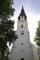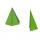Roof 7

The roof has the shape of a regular quadrangular pyramid with a base edge of 12 m and a height of 4 m. How many percent is folds and waste if in construction was consumed 181.4m2 of plate?

Result

p =  4.815 %

Solution:Leave us a comment of example and its solution (i.e. if it is still somewhat unclear...):Be the first to comment!To solve this example are needed these knowledge from mathematics:

Our percentage calculator will help you quickly calculate various typical tasks with percentages. Pythagorean theorem is the base for the right triangle calculator. See also our trigonometric triangle calculator.

Next similar examples:

1. Church roofThe roof of the church tower has the shape of a regular tetrahedral pyramid with base edge length 5.4 meters and a height 5 m. It was found that needs to be corrected 27% covering of the roof area. What amount of material will be required?
2. 4s pyramidRegular tetrahedral pyramid has a base edge a=17 and collaterally edge length b=32. What is its height?
3. TetrahedronCalculate height and volume of a regular tetrahedron whose edge has a length 18 cm.
4. TentPyramid-shaped tent has a base square with a side length of 2 m and a height 1.7 m. How many meters of canvas is nneded to make it if for a waste should be added 10%?
5. Lamp coneCalculate the surface of a lamp shade shaped of a rotary truncated cone with base diameter 32 cm and 12 cm and height 24 cm.
6. PyramidThe pyramid has a base rectangle with a = 6cm, b = 8cm. The side edges are the same and their length = 12.5 cm. Calculate the surface of the pyramid.
7. Triangle IRTIn isosceles right triangle ABC with right angle at vertex C is coordinates: A (-1, 2); C (-5, -2) Calculate the length of segment AB.
8. Triangle ABCIn a triangle ABC with the side BC of length 2 cm The middle point of AB. Points L and M split AC side into three equal lines. KLM is isosceles triangle with a right angle at the point K. Determine the lengths of the sides AB, AC triangle ABC.
9. Euclid 5Calculate the length of remain sides of a right triangle ABC if a = 7 cm and height vc = 5 cm.
10. Spruce heightHow tall was spruce that was cut at an altitude of 8m above the ground and the top landed at a distance of 15m from the heel of the tree?The double ladder shoulders should be 3 meters long. What height will the upper top of the ladder reach if the lower ends are 1.8 meters apart?If 5% more is gained by selling an article for Rs. 350 than by selling it for Rs. 340, the cost of the article is:We want to prove the sentence: If the natural number n is divisible by six, then n is divisible by three. From what assumption we started?Right isosceles triangle has an altitude x drawn from the right angle to the hypotenuse dividing it into 2 unequal segments. The length of one segment is 5 cm. What is the area of the triangle? Thank you.In an isosceles triangle ABC is |AC| = |BC| = 13 and |AB| = 10. Calculate the radius of the inscribed (r) and described (R) circle.The double ladder is 8.5m long. It is built so that its lower ends are 3.5 meters apart. How high does the upper end of the ladder reach?Given vector OA(12,16) and vector OB(4,1). Find vector AB and vector |A|.